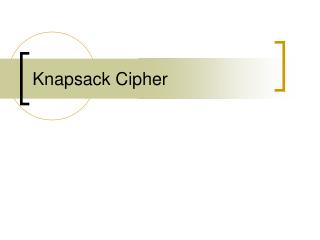DownloadDownload PresentationKnapsack Cipher

# Knapsack Cipher

Télécharger la présentation## Knapsack Cipher

- - - - - - - - - - - - - - - - - - - - - - - - - - - E N D - - - - - - - - - - - - - - - - - - - - - - - - - - -
##### Presentation Transcript

1. Knapsack Cipher

2. 0-1 knapsack problem • Given a positive integer C and a vector A=(a1,...,an) of positive integers, find a subset of the elements of A that sum to C; that is, find a binary vector M=(m1,...,mn) such that C=AM, or

3. Example of 0-1 knapsack problem • Let n=5, C =14, and A=(1,10,5,22,3). • Then M=(1,1,0,0,1) is a solution. • The knapsack problem is an NP-complete problem. • The best known algorithms for solving arbitrary instances of size n required O(2n/2) time and O(2n/4) space.

4. Special class of knapsack problem • a special class of knapsack problems, referred to as simple knapsack, that can be solved in linear time. • In a simple knapsack, the elements ai (i=1,...,n) are super increasing so that • This implies that

5. Example of simple knapsack problem • A’=(1,3,5,10,22) • A’ is a simple vector • snap(14, A’) gives the solution (1,1,0,1,0).

6. Algorithm for simple knapsack

7. Introduction • Merkle and Hellman proposed a scheme whose security depends on the difficulty of solving the 0-1 knapsack problem. • Merkle and Hellman show how to convert a simple knapsack into a trapdoor knapsack that is hard to solve without additional information.

8. Algorithm • First, a simple knapsack vector A’=(a1’,...an’) is selected. • This allows an easy solution to a problem C’=A’M. • Next, an integer u is chosen such that

9. Algorithm • Then an integer w is chosen such that gcd(u,w)=1, and the inverse w-1 of w mod u is computed using w-1=inv(w,u). • Finally, the vector A’ is transformed into a hard knapsack vector A=wA’ mod u; that is a1=w*ai’ mod u. • Now, solving C=AM is difficult.

10. Algorithm • With the knowledge of the trapdoor information w-1 and u, the problem can be transformed into the easy problem: • C’=w-1C mod u = w-1AM mod u = w-1(wA)M mod u =A’ M’ mod u = A’ M.

11. Public-Key system • public-key: A • secret-key: A’ • trapdoor information u and w-1 • A’=w-1A mod u • EA, DA denote the enciphering and deciphering; • C=EA(M)=AM • DA(C)=snap(w-1C mod u, A’)=M.

12. Example • Let A’=(1,3,5,10), u=20, and w=7. Then w-1=3. The simple vector A’ is transformed into the “hard” vector • A=(7*1 mod 20, 7*3 mod 20, 7*5 mod 20, 7*10 mod 20)=(7,1,15,10) • Let M=13, which is the binary vector (1,1,0,1)（二進位表示法） • Then C=EA(M)=7+1+10=18 • DA(C)=DA(18)=snap(3*18 mod 20, A’) =snap(14, A’)=(1,1,0,1)=13.

13. length of N • Merkle and Hellman originally suggested that using n=100 or more. • The best known algorithms for solving arbitrary instances of size n required O(2n/2) time and O(2n/4) space. • For n=100, 250=1015 • For n=200, 2100=1030

14. Example of

15. Graham-Shamir Knapsacks Graham and Shamir independently discovered a way of obscuring the super increasing property of trapdoor knapsacks. A Graham-Shamir trapdoor knapsack vector A’(a1’, ...,an’) has the property that each aji has the following binary representation: aj’=(Rj, Ij, Sj) • where Rj and Sj are long random bit strings, • Ij is a bit string of length n such that the jth high-order bits is 1 and the remaining n-1 bits are 0.

16. Graham-Shamir Knapsacks • Each random bit string Sj has log2n 0’s in its high-order bit position so that summing does not cause them to overflow into the area of the Ij’s. • Thus, a sum C’=A’M has the binary representation: • C’ =(R, M, S),where • Notice that the vector of bit strings(( In, Sn),...,(I1,S1))

17. Example of Graham-Shamir

18. Example of Graham-Shamir

19. Graham-Shamir • A trapdoor knapsack vector A’ is converted to a hard knapsack vector A as in the MerKle-Hellamn scheme;(by picking u and w and computing A=wA’ mod u). • A message M is enciphered by computing C’=EA(M)=AM. • C is deciphered by computing C’= w-1C mod u and extracting from C’ the bits representing M.